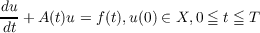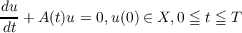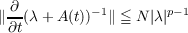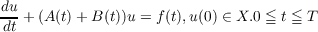#### Vol. 22, No. 3, 1967

 Download this articleFor screen For printingRecent Issues Vol. 325: 1 Vol. 324: 1  2 Vol. 323: 1  2 Vol. 322: 1  2 Vol. 321: 1  2 Vol. 320: 1  2 Vol. 319: 1  2 Vol. 318: 1  2Online Archive Volume: Issue:The Journal Subscriptions Editorial Board Officers Contacts Submission Guidelines Submission Form Policies for Authors ISSN: 1945-5844 (e-only) ISSN: 0030-8730 (print) Special Issues Author Index To Appear Other MSP Journals
The higher order differentiability of solutions of abstract evolution equations

### Ponnaluri Suryanarayana

Vol. 22 (1967), No. 3, 543–561
##### Abstract

In this paper, the regularity of the solution of the initial value problem for the abstract evolution equation(0.1)

and the associated homogeneous equation(0.2)

in a Banach space X is considered. Here u = u(t) and f(t) are functions from [0,T] to X and A(t) is a function on [0,T] to the set of (in general) unbounded linear operators acting in X.

Definition. u(t) is called a strict solution of (0.1) or (0.2) in (s,T] if

(i) u(t) is strongly continuous in the closed interval [s,T] and is strongly continuously differentiable in the semiclosed interval (s,T],

(ii) u(t) D(A(t)), the domain of A(t), for each t (s,T],

(iii) u(t) satisfies (0.1) resp. (0.2) in (s,T],u(s) coinciding with the given initial value at t = s. It is assumed that A(t) for each t [0,T] satisfies the following conditions.

(i) A(t) generates a semigroup exp(sA(t)) of operators analytic in the sector |arg s| < 𝜃,s0,0 < 𝜃 < π∕2,

(ii) For any complex number λ satisfying |arg λ| < π∕2 + 𝜃, 0 < 𝜃 < π∕2,(∂∕∂t)(λ + A(t))1 exists in the operator topology and that there exist constants N and ρ independent of t and λ with N > 0,0 ρ < 1 such thatThe main result proved in the paper can be stated as follows. If, in addition to the above assumptions, A(t)1 Cn+α[0,T] in the uniform operator topology, B(t), a bounded operator for each t [0,T] is of class Cn1+β[0,T], and f(t) Cn1+γ[0,T] in the strong topology, then the unique strict solution u(t) ofbelongs to the class Cn+δ[s0,T],s0 > 0 arbitrary, δ > 0 depending on α,β,γ and ρ. In this no assumption regarding the constancy of the domain D(A(t)) is made.

Primary: 34.95
##### Milestones